K_bMOM_Anatomy
In :
from warnings import simplefilter
simplefilter(action='ignore', category=FutureWarning)
simplefilter(action="ignore", category=RuntimeWarning)
from IPython.display import HTML
import random

def toggle_code(text_1="Show code", text_2="Hide code"):

rand = 'id'+str(random.randint(1,2**64))

html = """
<script>
function code_toggle_{random}() {{
var element = $('#{random}').parent().parent().parent().parent().parent().find('div.input') element.toggle() if(element.is(":hidden"))$('#{random}').text("{text_1}")
else
$('#{random}').text("{text_2}") }}$(document).ready(function() {{
code_toggle_{random}();
$('#{random}').parent().parent().find('div.prompt.output_prompt').text("") }}) </script> <a id="{random}" style="cursor: pointer;" onclick="javascript:code_toggle_{random}()">{text_1}</a> """.format(random = rand, text_1 = text_1, text_2=text_2) return HTML(html) toggle_code()  Out: Show code # K-bMOM ## Table of contents ### A. Context and bibliography¶ ### B. How K-bMOM works¶ #### 1. Initialization¶ #### 2. How to choose$B$and$S$¶ #### 3. Iteration¶ ### C. Use of K-bMOM¶ #### 1. Launch K-bMOM¶ #### 2. Review all information in output¶ ##### – centroids¶ ##### – labels¶ ##### – id_Qblock_init¶ ##### – convergence¶ ##### – scores¶ ##### – risk¶ ##### – Qb_centers¶ ##### – B¶ ##### – t¶ ## Context and bibliography K-bMOM is an algorithm that were first introduced by SAUMARD Adrien, BRUNET-SAUMARD Camille and GENETAY Edouard in februar 2020 in this article: https://arxiv.org/pdf/2002.03899.pdf K-bMOM is a clustering algorithm inspired from both K-means and Median-Of-Means (MOM). These two algorithms have advantages whose K-bMOM benefits from: on the one hand, Kmeans is an unsupervised clustering algorithm. On the other hand, it has been recently proved (2015) that MOM is a way better mean operator than the empirical mean because it is robust to outliers (it has also a better convergence rate). As a result, K-bMOM is indeed a robust and unsupervised clustering algorithm. We will describe K-bMOM in the next section. We are going to give in the following paragraphs some context clues. In principle, K-means is a clustering algorithm that clusterizes vector data in$\mathbb{R}^p$. More precisely, K-means searches for K points$\left( c_1,c_2,…,c_K\right) $(called centroids) that minimize this quantity$\text{Crit} := \frac{1}{n} \sum_{i=1}^{n} \underset{1\leq j \leq K}{\min} || x_i – c_j||^2$. Since essence of clustering is mostly the search for the « most relevant » summary of the data, K-means often fails to find the most relevant centroids. That is especially due to the criterion$\text{Crit}$. The optimization of this criterion leads to centroids that are, geometrically speaking, the « most relevant », and even if the geometrical optimum sometimes coincides with what one calls the « most relevant summary of the data », it often does not. A solution to this issue could come from the use of a better « mean operator » than the « empirical mean » in the criterion$\text{Crit}$. What is called « empirical mean » is namely to sum a bunch of data and to divide the result by the number of elements one has summed up (an example is given in the next paragraph; formally speaking, it is this type of operation:$ \frac{1}{n} \sum_{i=1}^{n}… $). Consequently, the recent theoretical discoveries about « estimating the mean-value of a probability distribution », such as the work about MOM, made K-bMOM possible. Let us explain a few things about some properties of « estimating the mean-value of a probability distribution ». The « empirical mean » of$n$observations$\left(x_1,x_2,…x_n \right)$of a random phenomenon is$\frac{1}{n} \sum_{i=1}^{n}x_i$. It is the most basic statistic that every one knows. For example: you take the size (in cm) of every close collegues of yours:$\left\{ 168,166,191,160,179 \right\}$. Then divide by the amount of data,$5$, and you get an average size of$ 1.728$cm (the empirical mean of the observed sizes is 172.8 cm). The empirical mean has been studied by mathematician since 18th century: • Abraham De Moivre found in 1738 that empirical mean gets closer and closer of the mean of a Bernoulli distribution as the number of observation grows. • After that, Pierre-Simon De Laplace proved in 1809 the first version of the central limit theorem that concerns a property of the empirical mean • One should wait the work of Alexander Khinchin published in 1929 to obtain a fully general theorem called « weak law of large numbers » (https://www.math.drexel.edu/~tolya/methodofmoments.pdf). This theorem proves that the empirical mean converges in probability to the mean-value of any random phenomenon as the number of observation increases. • But the endavour for a better understanding of empirical mean continued and Catoni proved in 2015 that the empirical mean suffers from a slow convergence rate without further assumptions on the observed phenomenon. He proved indeed that there exists some random phenomenon with a mean-value$\mu$and a finite variance$\sigma^2$for which the empirical mean operator has poor convergence rates (poor concentration rates) because the best one can insure is the Tchebychev inequality :$ \mathbb{P} \left( \lvert \frac{1}{n} \sum_{i=1}^{n}x_i-\mu \rvert >\epsilon \right) \leq \frac{\sigma^2}{\epsilon^2} $(http://www.numdam.org/article/AIHPB_2012__48_4_1148_0.pdf). The convergence rate above is not satisfying compared to the one achieved by MOM because$\frac{\sigma^2}{\epsilon^2}$vanishes polynomially quickly as$\epsilon$grows while the rate of MOM vanishes exponentially quickly as$\epsilon$grows. This property has been proved but Lugosi and al. in 2015 (https://projecteuclid.org/download/pdfview_1/euclid.aos/1479891632). Last but not least concerning the properties of MOM: it is robust to the presence of observations that are very far from the mean-value. This means that the MOM operator does still give output close to the mean-value of the interesting phenomenon while some observations do not follow the average behaviour. Inspired from these two estimators K-means and MOM, we proposed K-bMOM # How K-bMOM works ## 1. Initialization The principle of K-bMOM is to create$B$blocks from the dataset. So it does at the initialization : it will retain the centers of the block whose empirical risk is median to all blocks. Input : the dataset$\{x_1, . . . , x_n\}$,$B$the number of blocks and$S$size of blocks, with$S > K$1. Iterate from 1 until$B$blocks: (a) Select at random, uniformly and with replacement$S$datapoints (b) Proceed a kmeans++ initialisation on the block (c) Compute the empirical risk of the block 1. Select the centers from the block having the median empirical risk Output : Centers ## Example :¶ Let’s take a set of 600 data : In : from sklearn.datasets import make_blobs import matplotlib.pyplot as plt #To avoid the plt.show bug %matplotlib inline X, y = make_blobs(n_samples=600, centers=4, n_features=2, random_state=1) plt.scatter(X[:,0],X[:,1],1) plt.show() toggle_code()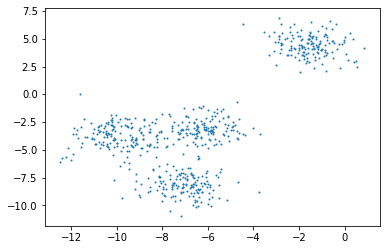Out: Show code ## A) Sampling_init The first step in initialization is to create blocks with the sampling_init function. It takes 3 hyperparameters:$B$the number of blocks,$S$their size and$K$number of clusters to find. On the following example,$B$=15 and$S$=20. We will explain later how to tune these values. (Note that the functions presented below have been simplified and adapted to the needs of the notebook) In : import numpy as np import random from scipy.spatial.distance import cdist from sklearn.cluster import KMeans def sampling_init(X,B,S,K,random_state,n_local_trials=1): ''' # Initialisation function: create nbr_blocks blocks, initialize with a kmeans++, compute a partition # X : dataset # B : number of blocks # s : number of data per block # K : number of clusters ''' # variables that are only used in this tutorials to explain how it works all_blocks_before_clustering = [] # body of code init_blocks = *B # instanciation of kmeans++ x_squared = X**2 x_squared_norms = x_squared.sum(axis=1) # Blocks creation for i in range(B): idx = random.choices(np.arange(X.shape),k = int(S)) all_blocks_before_clustering.append(idx) kmeans_obj = KMeans(n_clusters=K, random_state=random_state, n_init=n_local_trials, init='k-means++') kmeans_obj.fit(X=X[idx,:]) centers = kmeans_obj.cluster_centers_ km_dist = cdist(X[idx,:],centers) km_labels_ = km_dist.argmin(axis=1) init_blocks[i] = [[j for j,x in zip(idx,km_labels_) if x==k] for k in range(K)] return init_blocks,all_blocks_before_clustering  In : K = 4 B = 15 S = 20 nb_columns = 5 nb_rows = 3 seed = 11061109 random.seed(seed)  So, let’s execute sampling_init In : all_blocks_after_init_step, all_blocks_before_clustering = sampling_init(X,B=B,S=S,K=K,random_state=seed)  For example, here’s the 1st block In : all_blocks_after_init_step  Out: [[255, 281, 96], [150, 313, 179, 498, 527], [308, 554, 562, 373, 40], [396, 480, 70, 597, 386, 306, 51]] Here’s the 2nd block In : all_blocks_after_init_step  Out: [[443, 28, 66, 24, 43], [372, 536, 279], [111, 588, 11, 472, 430, 414], [107, 91, 524, 9, 57, 474]] Here’s the 8th block In : all_blocks_after_init_step  Out: [[69, 101, 234, 150, 524], [53, 529, 149, 149, 391, 450, 164, 45], [316, 219, 133], [209, 107, 259, 431]] Now, let’s display the plot of a block : In : from matplotlib.cm import get_cmap one_block_ = all_blocks_before_clustering plt.plot(X[one_block_][:,0],X[one_block_][:,1],'.', markersize=10) plt.title("The plot of a block") plt.show() toggle_code()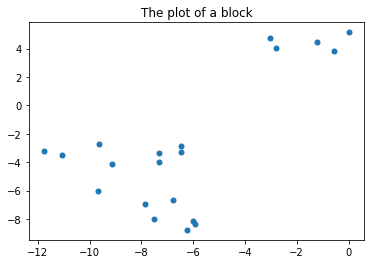Out: Show code In : one_block_ = all_blocks_after_init_step cmap = get_cmap('tab20') for cluster in range(K): colors = cmap((cluster)) plt.plot(X[one_block_[cluster]][:,0],X[one_block_[cluster]][:,1],'.',c=colors, markersize=10) plt.title('The same block after k-means++ initialization') plt.show() toggle_code()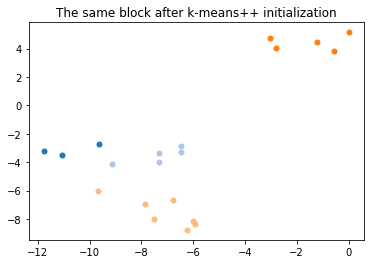Out: Show code That was for a single block, but keep in mind that there are 15 of them: In : marge = 1 prop = 2 fig, axs = plt.subplots(nb_rows, nb_columns,figsize=(prop*5,prop*3)) xlim_ = (np.min(X[:,0])-marge,np.max(X[:,0])+marge) ylim_ = (np.min(X[:,1])-marge,np.max(X[:,1])+marge) for row in range(nb_rows): for column in range(nb_columns): one_block_ = all_blocks_before_clustering[column+row*nb_columns] axs[row,column].plot(X[one_block_][:,0],X[one_block_][:,1],'.') axs[row,column].set_xlim(xlim_) axs[row,column].set_ylim(ylim_) axs[row,column].set_title(str(column+row*nb_columns+1)+': ') plt.subplots_adjust(wspace = 0.5,hspace = 0.6) plt.suptitle('All blocks of data randomly uniformly picked among the dataset with replacement', fontsize=15) plt.show() toggle_code()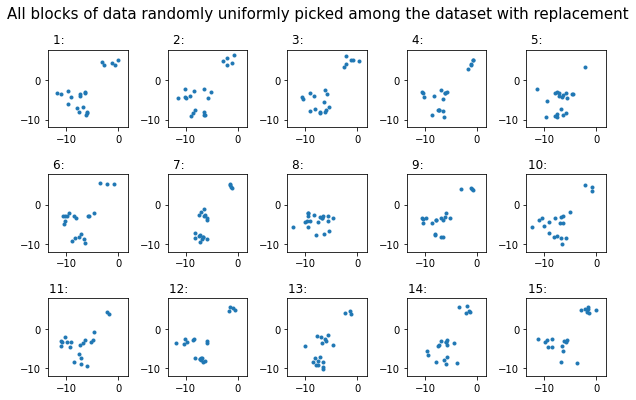Out: Show code In : cmap = get_cmap('tab20') fig, axs = plt.subplots(nb_rows, nb_columns,figsize=(prop*5,prop*3)) for row in range(nb_rows): for column in range(nb_columns): one_block_ = all_blocks_after_init_step[column+row*nb_columns] for cluster in range(K): colors = cmap((cluster)) axs[row,column].plot(X[one_block_[cluster]][:,0],X[one_block_[cluster]][:,1],'.',c=colors) axs[row,column].set_xlim(xlim_) axs[row,column].set_ylim(ylim_) plt.xlim(xlim_) axs[row,column].set_title(str(column+row*nb_columns+1)+': ') plt.suptitle('All blocks after k-means++ initialization', fontsize=20) plt.subplots_adjust(wspace = 0.5,hspace = 0.6) plt.show() toggle_code()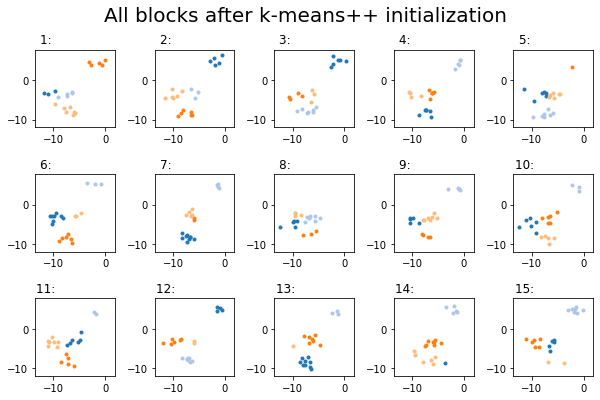Out: Show code ## B) Qrisk Then we will compute the risk for each block. First, the function within_var, computes the inertia of each cluster of a block In : def within_var(one_block,X): ''' # Function which returns a list of within inertia per cluster for the given block # one_block : dictionnary of the subsample of one block according to the clusters ''' n_b = 0 var_b = *K for key , val in enumerate(one_block): X_block = X[val,:] var_b[key] = len(val)*np.sum(np.var(X_block,axis=0))/S return var_b  In : within_var(one_block_,X)  Out: [0.2702099634777766, 0.31298671791910315, 0.45981505060761807, 0.23046722906471157] Then, Qrisk computes the risk of each block and returns the block that got the median risk In : quantile = 0.5 # this corresponds to taking the median def Qrisk(all_blocks,X): ''' # Function which computes the sum of all within variances and return: - Q_risk = the q-quantile block risk - Q_id = id of the associated q-quantile block - dict_within[Q_id] = list of the within variances of the selected bloc parameters  . all_blocks : output of sampling_all_blocks . X : matrix of datapoints ''' dict_within = *B Qb_risks = *B for key, one_block_ in enumerate(all_blocks): dict_within[key] = within_var(one_block_,X) Qb_risks[key] = sum(dict_within[key]) risks_argsort = np.argsort(np.array(Qb_risks),kind="mergesort") quantile_index = risks_argsort[round(quantile*B)-1] quantile_value = Qb_risks[quantile_index] return dict_within[quantile_index],all_blocks[quantile_index],quantile_index  Let’s take a look of all risks : In : cmap = get_cmap('tab20') fig, axs = plt.subplots(nb_rows, nb_columns,figsize=(prop*5,prop*3)) xlim_ = (np.min(X[:,0])-3,np.max(X[:,0])+3) for row in range(nb_rows): for column in range(nb_columns): one_block_ = all_blocks_after_init_step[column+row*nb_columns] for cluster in range(K): colors = cmap((cluster)) axs[row,column].plot(X[one_block_[cluster]][:,0],X[one_block_[cluster]][:,1],'.',c=colors) axs[row,column].set_title('{}: risk={}'.format(column+row*nb_columns+1,round(sum(within_var(all_blocks_after_init_step[column+row*nb_columns],X)),2))) axs[row,column].set_xlim(xlim_) axs[row,column].set_ylim(ylim_) plt.xlim(xlim_) plt.suptitle('All risks', fontsize=20) plt.subplots_adjust(wspace = 0.5,hspace = 0.6) plt.show() toggle_code()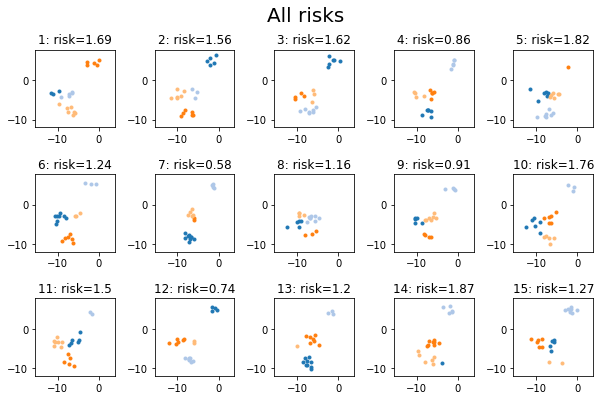Out: Show code In : dict_within, Q_block, id_of_the_Q_block = Qrisk(all_blocks_after_init_step,X) phrase = 'The {}th block was the one to achieve the median value of the risk among all blocks' print(phrase.format(id_of_the_Q_block+1)) print('median risk = {}'.format(round(sum(dict_within),2))) toggle_code()  The 15th block was the one to achieve the median value of the risk among all blocks median risk = 1.27  Out: Show code We can also see that the 8th block is an occurence of an unlikely event : no data from the cluster on the top right has been picked contrary to the other clusters (as you can see on the plot below). In : block_ = all_blocks_after_init_step fig = plt.figure(figsize=(prop*7,prop*2)) ax1 = fig.add_subplot(1, 2, 1) ax2 = fig.add_subplot(1, 2, 2, sharex=ax1) ax1.scatter(X[:,0],X[:,1],3,c="Blue") ax1.set_title('Complete dataset') for cluster in block_: ax2.scatter(X[cluster][:,0],X[cluster][:,1],3,c="Blue") ax2.set_title('8th block') plt.ylim(ylim_) plt.xlim(xlim_) plt.suptitle('', fontsize=20) plt.show() toggle_code()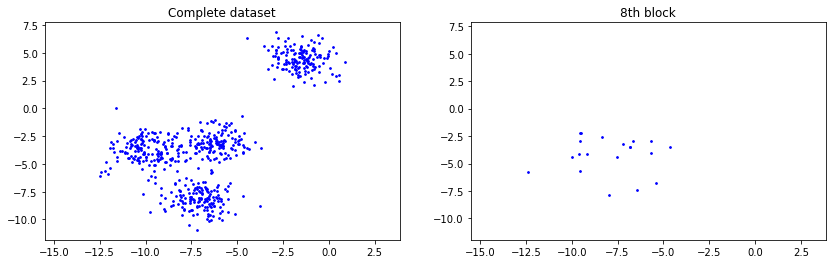Out: Show code Fortunately, this kind of particular cases will not be retained because their risk will not be median. Let’s plot the median block : In : cmap = get_cmap('tab20') plt.subplots(figsize=(8,5)) for cluster in range(K): colors = cmap((cluster)) plt.plot(X[Q_block[cluster]][:,0], X[Q_block[cluster]][:,1], '.', c=colors, markersize=10) plt.xlim(xlim_) plt.ylim(ylim_) plt.title('The block with the median risk') plt.show() toggle_code()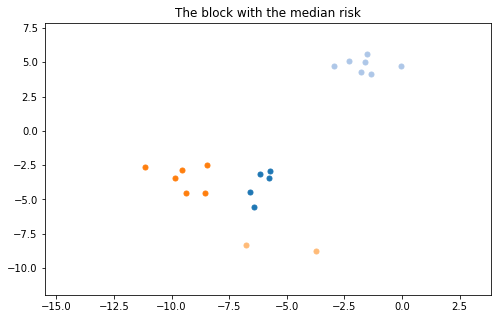Out: Show code ## C) Update_loop At this point, initialization is almost complete. All we have to do now is to determine the centers and the partition. The update_loop function (which we’ll talk about later) takes care of finalization. In : def Qb_centers(Q_block,X): ''' #compute the mean vector of each cluster in the q-quantile block and return it ''' centers_ = [] for k in range(K): center_k = X[Q_block[k],:].mean(axis=0) centers_.append(center_k) return np.array(centers_) def update_loop(X,Qb): ''' # Qb : Q-quantile block # Q_block_list_of_within_var : within inertia of the qq block ''' # updates centers centers = Qb_centers(Qb,X) # retrieve partition of data D_nk = cdist(XA=X,XB=centers) partition_array = D_nk.argmin(axis=1) partition_list = [] for k in range(K): partition_list.append(np.where(partition_array == k).tolist()) # compute empirical risk D_nk_min_rows = D_nk[[np.arange(X.shape).tolist(),partition_array.tolist()]] return centers, partition_array,partition_list  In : centers, partition,partition_list = update_loop(X,Q_block)  That’s it, the initialization is complete, let’s display the result: In : plt.figure(figsize=(15,10)) plt.scatter(X[:,0],X[:,1],10,c=tuple(partition)) plt.scatter(centers[:,0],centers[:,1],100,c="red") plt.title("Initialization completed : initial clustering", fontsize=30) plt.autoscale() plt.show() toggle_code()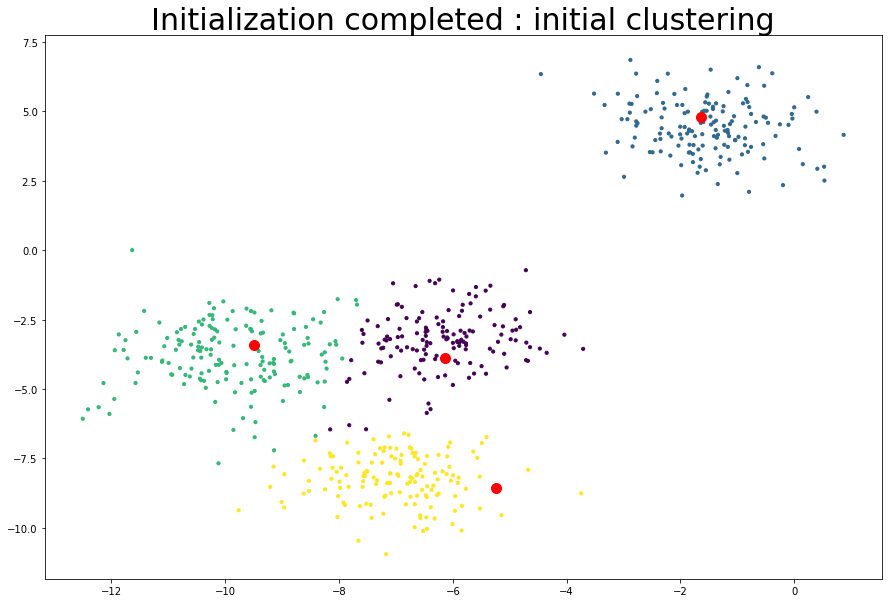Out: Show code Below is the initialization code present in the K-bMOM class : In : def initialisation_without_init_centers(self,X): # Initialisation step : initialisation per block: sampling B blocks and init via kmeans++ init_blocks = self.sampling_init(X) # compute empirical risk among blocks and select the Q-quantile-block Q_within, Q_b = self.Qrisk(init_blocks,X) # update all the global variables self.update_loop(X,Q_b,Q_within) # save results self.id_Qblock_init = Q_b #self.res_Qb_risk.append(Q_risk)  ## 2. How to choose$B$and$S$Just before cell In , we told you that we were going to explain how to find the$B$and$S$values. They are calculated using mathematical equations. In : from math import log, ceil, floor, inf def block_size_max(n_sample,n_outliers): ''' Function which fits the maximum size of blocks before a the breakpoint prms n_sample: nb of data n_outlier: nb of outliers ''' if n_outliers == 0: bloc_size_max = inf else: bloc_size_max = log(2.)/log(1/(1- (n_outliers/n_sample))) return floor(bloc_size_max) def minimal_number_of_blocks(n_sample,n_outliers,b_size=None,alpha=0.05): ''' Function which fits the minimum nb of blocks for a given size t before a the breakpoint prms n_sample: nb of data n_outlier: nb of outliers b_size = bloc_size alpha : threshold confiance ''' if n_outliers/n_sample >= 0.5: print('too much noise') return() else: if n_outliers == 0 : return 1 elif b_size is None: t = bloc_size(n_sample,n_outliers) else: t = b_size bloc_nb_min = log(1/alpha) / (2* ((1-n_outliers/n_sample)**t - 1/2)**2) return ceil(bloc_nb_min)  First you have to choose the size of the blocks, then the number of blocks. We realize that the bigger the size of a block is, the bigger the number of blocks must be, so in order to avoid too much complexity, it is better to choose a block size smaller than the maximum allowed. Look at the curve below to see how$B$behaves as$S$grows. In : S = block_size_max(n_sample=600,n_outliers=5) list_block_size = [] list_nb_blocks = [] abscisse = np.round(np.linspace(K,S-2,100)) ticks = np.round(np.linspace(K,S-2,10)) if S < inf: for k in abscisse: list_block_size = list_block_size + [k] list_nb_blocks = list_nb_blocks + [ minimal_number_of_blocks(n_sample=600,n_outliers=5,b_size=k,alpha=0.05) ] plt.plot(list_block_size,list_nb_blocks) plt.xticks(ticks) plt.xlabel('S : Block sizes') plt.ylabel('B : Number of Blocks') plt.show() toggle_code()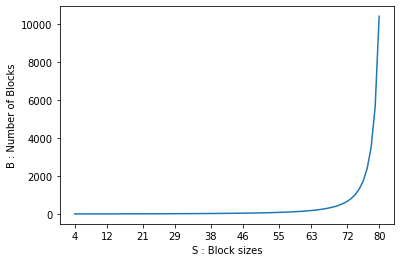Out: Show code In : block_size_max(n_sample=600,n_outliers=5)  Out: 82 (Please note that the parameter n_outliers, is actually an estimated majoration of the amount of outliers in your dataset) The maximum size of a block should be 82, but as you have understood, we will take a lower value. In this case you can take$S$equal to 60. (For our example we used$S$=20 for pedagogical reasons) In : minimal_number_of_blocks(n_sample=600,n_outliers=5, b_size=20)  Out: 13 Now you can run minimal_number_of_blocks. 13 is the minimum value you can use, and that’s why we chose$B$=15 in our previous example. ## 3. Iteration In reality, the iterations are very close to the initialization process. Input : the dataset$\{x_1, . . . , x_n\}$,$B$the number of blocks and$S$size of blocks ($S$>$K$) Main Loop : WHILE the$stop Criterion$isn’t reached or$iter$<$iter$max: 1. Create$B$blocks of the data of size$S$randomly and uniformly with replacement 2. In each block, assign each datapoint to its closest centroid. 3. Keep the block with the median empirical risk 4. Calculate the new centers Output : Partition A CHOISIR Input : the dataset$\{x_1, . . . , x_n\}$,$B$the number of blocks and$S$size of blocks ($S$>$K$) Main Loop : WHILE the$stop Criterion$isn’t reached or$iter$<$iter$max: 1. Iterate from 1 until$B$blocks : • Create a block with size$S\$ randomly and uniformly with replacement
• Assign each datapoint to its closest centroid.

2. Keep the block with the median empirical risk

3. Compute the new centers

Output : Partition

The only major difference is the construction of the blocks.
They are built using the sampling_all_blocks function which is very close to sampling_init.
However, thanks to the nb_min_repr_by_cluster parameter, each cluster in each block will have at least nb_min_repr_by_cluster data. This value is set to 1 by default.

In :
def sampling_all_blocks(self, X, nb_min_repr_by_cluster=1):#,nbr_blocks,weighted_point,cluster_sizes):
'''
# Function which creates nbr_blocks blocks conditionally to the partition_dict and return a dictionary
# per block of the ids of selected  data per cluster and per bloc
#
#     nbr_blocks      : number of blocks to be created
#     weighted_point : a dictonnary of clusters containing the lists of weights
#     cluster_sizes   : vector containing the size of each clusters
'''
dict_blocks = *B
for i in range(B):
# to ensure there are K categories in each block run that part, one can go through the first piece of code, else run 'else'
list_of_points = []
if nb_min_repr_by_cluster is not None:
for k in range(K):
list_of_points = list_of_points + random.choices(np.array(self.partition_dict[k]), k=nb_min_repr_by_cluster)
idx_indep = random.choices(np.arange(X.shape), k=S-nb_min_repr_by_cluster*K)
else:
idx_indep = random.choices(np.arange(X.shape), k=S)
idx = list_of_points + idx_indep

km_dist    = cdist(X[idx,:],centers)
km_labels_ = km_dist.argmin(axis=1)
dict_blocks[i] = [[j for j,x in zip(idx,km_labels_) if x==k] for k in range(self.K)]

return dict_blocks


# Use of K-bMOM

In :
import numpy as np
from Modules.KbMOM_v8_4_before_5 import KbMOM # KbMOM stands for K-bootstrap Median-Of-Means
from sklearn.datasets import make_blobs
import matplotlib.pyplot as plt

#To avoid the plt.show bug
%matplotlib inline

X, y = make_blobs(n_samples=600, centers=4, n_features=2, random_state=1)
plt.scatter(X[:,0],X[:,1],1)
plt.show()## 1. Launch K-bMOM

In :
instance_KbMOM = KbMOM(K=4,X=X,coef_ech=20,nbr_blocks=15,nb_min_repr_by_cluster=1)

In :
results_KbMOM = instance_KbMOM.fit(X=X)

In :
results_KbMOM

Out:
{'centroids': array([[ -4.76718795,  -1.74560175],
[  0.40622341,   2.93533294],
[-10.53661635,  -4.21951699],
[ -7.20441682,  -9.07633508]]),
'labels': array([3, 0, 3, 2, 1, 0, 0, 1, 0, 2, 1, 3, 0, 0, 1, 1, 2, 3, 1, 1, 3, 2,
1, 3, 1, 3, 3, 3, 1, 2, 1, 3, 1, 1, 3, 1, 2, 3, 0, 0, 1, 1, 3, 1,
1, 2, 2, 1, 2, 1, 0, 3, 0, 0, 0, 3, 1, 2, 1, 2, 2, 2, 3, 2, 3, 2,
1, 1, 2, 2, 3, 0, 0, 3, 3, 0, 3, 3, 1, 3, 2, 2, 3, 1, 2, 2, 2, 3,
1, 2, 1, 2, 0, 0, 3, 2, 2, 2, 1, 3, 2, 2, 0, 1, 2, 0, 3, 2, 1, 2,
2, 3, 1, 2, 3, 0, 3, 1, 1, 2, 1, 2, 2, 1, 0, 0, 3, 3, 0, 0, 2, 3,
3, 3, 3, 0, 0, 2, 1, 1, 0, 0, 3, 2, 1, 1, 3, 2, 1, 0, 2, 0, 2, 0,
3, 3, 2, 2, 2, 3, 2, 1, 2, 0, 0, 1, 1, 3, 2, 3, 1, 2, 3, 2, 2, 3,
3, 1, 1, 0, 1, 3, 2, 3, 2, 2, 2, 3, 2, 0, 1, 1, 1, 3, 0, 3, 1, 0,
1, 2, 1, 3, 1, 1, 3, 2, 3, 1, 3, 2, 3, 2, 1, 1, 3, 0, 1, 0, 0, 3,
3, 0, 0, 2, 1, 2, 3, 2, 2, 3, 3, 2, 3, 0, 2, 1, 1, 3, 0, 0, 3, 2,
2, 2, 2, 3, 1, 0, 2, 3, 2, 0, 2, 3, 0, 2, 2, 3, 1, 2, 1, 1, 0, 0,
3, 2, 1, 3, 0, 1, 0, 2, 2, 1, 0, 1, 3, 2, 0, 0, 1, 2, 3, 2, 3, 2,
3, 1, 1, 1, 1, 2, 0, 3, 1, 3, 2, 0, 0, 1, 3, 1, 3, 0, 2, 0, 3, 3,
1, 2, 3, 1, 1, 2, 2, 3, 3, 1, 1, 2, 3, 0, 0, 3, 3, 0, 2, 3, 2, 1,
0, 0, 1, 2, 3, 3, 0, 0, 3, 3, 3, 0, 3, 2, 2, 1, 0, 2, 0, 3, 1, 3,
3, 2, 0, 1, 0, 2, 0, 2, 0, 1, 3, 2, 2, 1, 3, 1, 0, 1, 2, 2, 0, 1,
2, 1, 0, 1, 1, 2, 2, 3, 0, 0, 2, 1, 3, 0, 1, 2, 3, 0, 2, 2, 0, 2,
3, 3, 2, 3, 1, 1, 1, 3, 2, 1, 2, 0, 3, 1, 0, 1, 2, 1, 3, 3, 2, 1,
1, 0, 0, 3, 0, 1, 3, 2, 2, 2, 3, 0, 3, 2, 0, 3, 1, 1, 1, 0, 3, 0,
3, 1, 3, 1, 1, 2, 2, 1, 1, 1, 0, 2, 2, 3, 2, 1, 2, 2, 0, 0, 2, 1,
3, 0, 1, 2, 0, 1, 1, 2, 2, 1, 3, 3, 2, 3, 3, 1, 1, 3, 2, 1, 0, 0,
2, 0, 2, 2, 0, 1, 1, 0, 0, 1, 0, 2, 3, 2, 0, 1, 0, 2, 2, 0, 3, 3,
2, 3, 3, 2, 0, 0, 2, 1, 1, 2, 3, 0, 0, 1, 3, 2, 3, 2, 2, 3, 3, 0,
3, 0, 0, 2, 2, 3, 0, 3, 0, 2, 2, 1, 2, 0, 2, 2, 0, 3, 0, 0, 3, 0,
2, 0, 3, 1, 1, 2, 1, 0, 2, 0, 2, 1, 1, 2, 0, 3, 3, 2, 2, 0, 3, 0,
0, 1, 3, 3, 2, 2, 3, 0, 1, 2, 0, 3, 1, 1, 2, 1, 3, 2, 0, 1, 0, 2,
0, 1, 1, 3, 1, 1]),
'id_Qblock_init': [[368, 8, 582, 530, 581, 581, 45, 164],
[28, 15, 207, 318],
[156, 431, 231, 241, 102, 427, 156],
],
'convergence': [None,
None,
1.654389994652857,
1.6724332851540002,
1.6098389737594274,
1.5780469038491978,
1.642370690499925,
1.5862243066642197,
1.6702825273687074,
1.6303510797913323,
1.7282894321499624],
'scores': array([4.81298029, 3.04654639, 3.34368445, 4.56279977, 2.03736067,
4.41556365, 3.61051846, 2.82775401, 3.72614243, 5.45401034,
2.4970349 , 5.46312105, 1.9852819 , 4.24218768, 2.22569067,
3.7760575 , 3.85259275, 6.32388233, 2.53614236, 1.85671006,
3.20843581, 4.04395517, 1.80641549, 5.05459253, 2.04069593,
4.01648993, 4.06611801, 3.63158607, 3.11968454, 5.41857847,
2.63116413, 2.33777097, 3.41623653, 1.84411811, 3.99028352,
2.17802384, 0.76058673, 4.70707056, 1.30387227, 1.88828279,
1.51823015, 1.87298802, 3.28689955, 2.36654242, 2.17253406,
2.22868104, 4.57116708, 2.64098134, 3.51212058, 2.59126555,
1.79990316, 4.58145613, 1.67586793, 3.57299956, 1.97172399,
3.15168398, 3.58438956, 2.80482031, 2.27707694, 3.94951441,
3.87586045, 3.26206843, 5.44536129, 4.26621517, 1.84006023,
1.87197475, 4.55449033, 3.20948132, 1.38759575, 1.51274799,
4.51552892, 3.9354732 , 1.78859314, 4.75978304, 4.66024868,
3.08568869, 3.63929269, 3.99364765, 3.18957784, 3.13110307,
2.10483774, 2.71809947, 3.17654082, 3.56357042, 2.81016314,
3.43042414, 3.01427362, 4.45295862, 3.47089852, 3.73043692,
3.63604216, 2.12097791, 2.07174052, 2.0294398 , 3.96356334,
3.28085573, 2.580317  , 3.71672912, 2.24640187, 4.36090979,
4.20519477, 2.72628676, 1.55106067, 2.23728845, 3.07250053,
4.69002104, 3.53086362, 0.99776329, 1.49131212, 3.771639  ,
2.78219955, 4.75906288, 2.25810023, 3.04523666, 4.10076258,
0.95425209, 4.97393301, 2.24768089, 3.84399751, 3.43452782,
3.07339363, 3.29542077, 4.59718718, 1.39401775, 2.56840684,
2.42057383, 2.34597261, 3.84859808, 1.14677227, 2.66180508,
2.16061828, 4.72014459, 4.04342516, 4.7643937 , 3.09809564,
1.7550656 , 4.62146239, 2.92874094, 3.39132108, 1.6281013 ,
2.0485337 , 2.97669207, 3.41130415, 2.93902095, 1.9759213 ,
3.7894485 , 1.38133641, 1.92210397, 3.45545993, 3.5592898 ,
2.84736499, 3.00817412, 2.66357665, 1.10712938, 4.26185738,
3.62734862, 5.01504236, 3.08053002, 3.86968467, 4.44142536,
3.66804541, 2.26854258, 2.07104191, 0.48187624, 4.24506526,
1.95183164, 2.79797916, 4.38447958, 3.3073198 , 3.9969354 ,
3.84449384, 4.28812847, 5.00940397, 3.87216638, 2.75831533,
4.9819218 , 5.41074039, 2.18166127, 1.69982501, 3.51021326,
3.67878967, 4.18028723, 3.48258812, 4.24925107, 3.85371187,
3.0134756 , 1.435005  , 3.31354165, 4.87486129, 0.96335746,
2.63668587, 1.9310027 , 2.34744567, 3.15720517, 1.43300076,
2.74192285, 3.62033822, 2.03770129, 2.43801566, 1.64175543,
1.93018227, 4.17682923, 2.48413415, 1.15368864, 4.2703346 ,
2.52434606, 4.93477606, 4.15968057, 3.88439544, 3.41614793,
5.48950386, 3.47871155, 1.90001751, 2.10575441, 3.15551842,
3.23742482, 2.51128938, 0.9271767 , 2.83891508, 1.87787951,
4.91292333, 2.1043694 , 2.64361794, 0.86373125, 3.80152526,
2.37334785, 1.54932106, 3.83459612, 2.68312199, 4.20139129,
4.32762705, 6.87603638, 4.2425547 , 3.48729495, 2.58417995,
1.91513945, 1.41074453, 3.59016418, 2.20334484, 1.55880224,
5.00837076, 3.5042996 , 2.96869774, 2.98050898, 2.57588024,
4.15572865, 2.52186863, 2.13137422, 3.62116888, 4.43002137,
3.64342131, 3.95348196, 1.85446722, 3.57333733, 4.64604243,
3.02385119, 2.86068683, 5.76611987, 2.54919302, 3.30155318,
1.77547988, 1.91369474, 4.22236622, 2.50449571, 4.20867857,
3.84015053, 2.56423324, 3.45804827, 1.83034986, 1.7357696 ,
1.28423352, 4.39247307, 3.82788247, 1.18163427, 2.16917253,
2.87713878, 4.69481173, 1.79923223, 3.63515308, 1.82119153,
2.19584424, 3.95599555, 4.09114192, 3.83481464, 3.69355471,
3.84107947, 3.43432014, 2.88599246, 3.43455972, 2.06096349,
3.45737778, 1.63629688, 3.01655278, 2.26466663, 2.76334164,
3.73881388, 5.17617973, 4.24820492, 2.70439238, 2.8994836 ,
2.33647627, 3.50490799, 3.76942159, 1.3113484 , 5.18298918,
1.5554214 , 2.1798794 , 2.55183663, 4.42449731, 3.05533205,
4.09063743, 3.90970584, 2.97751085, 1.93366202, 3.40476747,
1.33247257, 3.81897045, 2.49905541, 2.23656646, 2.46440531,
3.43525814, 4.75501993, 2.51651437, 3.91426298, 4.74251709,
1.65469586, 3.00204076, 3.08350158, 4.27465234, 1.94814321,
3.41215954, 2.21467247, 1.09571216, 2.72951887, 4.69885607,
3.7103279 , 4.1632198 , 4.62290383, 3.65145211, 4.25735988,
4.54166989, 1.7578717 , 4.17785964, 3.91169997, 2.75160143,
1.75635877, 4.9328937 , 0.92169823, 4.52986308, 3.8025746 ,
1.86585839, 3.45849763, 4.73928413, 2.82809897, 1.25398795,
1.71505485, 3.00166666, 0.13894817, 3.76015015, 2.7729513 ,
1.21829455, 3.09176533, 4.70914426, 5.4911195 , 4.14412552,
4.44408247, 5.16291349, 1.59354119, 4.38841925, 2.08773012,
1.91312076, 1.9833061 , 3.22918395, 2.49083827, 3.07459457,
1.43674323, 2.61944424, 2.47141153, 2.03467715, 1.00742573,
4.37614432, 4.8160156 , 2.73693785, 2.45529061, 1.38570553,
3.071245  , 4.72669019, 2.81457916, 3.99631738, 2.6553189 ,
2.05579188, 3.35984016, 3.1922675 , 2.98089808, 2.73155944,
2.67450541, 4.86926724, 2.90482874, 2.73030753, 1.48175952,
1.05071558, 1.46278091, 1.9077626 , 2.28169983, 2.4356121 ,
2.00016823, 2.21958809, 2.93421074, 4.64528104, 1.32186797,
0.63885614, 1.96322243, 2.46991797, 2.70896433, 2.37666258,
3.51394252, 2.29634245, 3.55614117, 2.25616722, 2.60978938,
4.20211445, 4.02787786, 1.44598667, 2.36589331, 2.42848631,
3.10428086, 4.37320982, 3.10050919, 5.14177887, 2.8273463 ,
5.4371452 , 3.59089155, 1.1033163 , 4.27264122, 3.78111448,
2.442504  , 4.69987817, 3.40364869, 4.68775561, 1.41141875,
4.97144821, 1.18735522, 1.78467466, 2.23112663, 3.3669427 ,
2.87401154, 3.02297277, 1.08449145, 3.56596218, 1.32690322,
1.57344428, 2.95905109, 2.44597591, 4.18086769, 4.24957566,
2.7049201 , 3.21815841, 5.47485637, 1.93254275, 4.76641721,
2.92373049, 3.52341762, 3.13377603, 2.14903652, 2.43450023,
3.54444911, 2.46473127, 1.20285089, 2.55051784, 6.6883597 ,
3.12706734, 3.63919967, 3.27451633, 1.17242147, 3.656983  ,
2.14509144, 4.68725201, 2.63667592, 3.53656058, 4.59304796,
0.98060931, 3.69530827, 1.26651618, 2.82862821, 3.86569008,
1.31595478, 2.7927012 , 2.39728277, 4.29420821, 3.3484728 ,
1.35073115, 2.04458725, 1.38837715, 2.19471982, 2.61024577,
3.38790131, 3.72248312, 4.47048338, 4.17707033, 3.93007697,
0.71753869, 4.14803131, 3.56711188, 5.02090329, 2.48022616,
4.4226052 , 5.20555697, 2.88916465, 4.08268974, 3.66723827,
1.57443722, 1.98714142, 2.2460818 , 2.99398277, 1.04337766,
2.77225666, 3.83732493, 2.00575259, 2.35443106, 2.05380337,
3.19766894, 3.18925942, 4.03673109, 2.99994553, 3.80286389,
3.63481325, 4.23026336, 3.30080819, 4.05081367, 3.55416699,
4.62498898, 4.55468667, 2.18065858, 4.27434643, 1.40899794,
3.67482126, 2.00517241, 4.95040148, 3.74793221, 2.63535333,
2.93121719, 1.92540572, 2.06447319, 2.05594348, 1.7082816 ,
4.8869032 , 4.73391624, 3.64687132, 3.89726532, 3.59435873,
3.07753857, 2.35354769, 3.38200653, 1.94026973, 1.81218776,
3.54966486, 3.04498682, 1.51061509, 2.68263823, 4.28532303,
3.86550884, 3.41292179, 3.65728968, 1.56353351, 2.67838202,
3.53746881, 3.8660898 , 3.65852401, 3.71524589, 4.13925574,
1.45784815, 2.43967968, 4.67257681, 1.57497865, 3.63594379,
2.79690806, 0.62286986, 3.01415901, 3.52065192, 1.87640299,
2.58242996, 3.018184  , 2.72604612, 0.52129954, 2.40231325,
1.6957612 , 2.48599553, 2.51493593, 4.01864592, 2.56760018,
1.65322574, 2.39265276, 3.51116597, 3.47765047, 3.42391577,
2.53303477, 3.42846678, 2.27395245, 3.79206427, 3.01188995]),
'risk': [3.774319440701109,
2.2001900434173383,
2.0041008174964574,
1.979601468849155,
2.1701678163427958,
2.255261450545043,
2.138029232218562,
2.360549995710217,
1.9543611108563739,
2.0907674140677286,
4.560213657366115],
'Qb_risk': [1.7500958670003555,
1.6694033275691018,
1.6567451287708095,
1.591205002642679,
1.6172415178284425,
1.6947976433818026,
1.5329924222850775,
1.6123231040321695,
1.645813941293566,
1.6170883547338206,
1.5783578647217495],
'Qb_centers': [array([[-7.10057907, -3.61362142],
[-1.83724548,  5.26348224],
[-9.43803981, -3.00970852],
[-5.1450899 , -9.54492198]]), array([[ -5.98287145,  -3.09665204],
[ -2.27986446,   4.84117782],
[-10.18453369,  -3.16584064],
[ -7.49531724,  -8.13852482]]), array([[ -6.00059938,  -3.51085505],
[ -1.15395686,   4.39244479],
[-10.09783712,  -3.5665143 ],
[ -6.78742338,  -8.38369569]]), array([[ -6.11781281,  -2.91514716],
[ -1.41984143,   4.78518195],
[-10.34678315,  -4.05487162],
[ -6.87361637,  -8.17011308]]), array([[ -6.93595347,  -3.20166746],
[ -1.09694799,   4.5273377 ],
[-10.4909522 ,  -4.42985701],
[ -6.76436449,  -8.15928559]]), array([[-5.92732468, -3.23120499],
[-2.03038093,  4.75705775],
[-9.9075231 , -2.77895483],
[-6.76082864, -8.30484026]]), array([[ -6.7744647 ,  -2.81848195],
[ -1.45193924,   4.56236768],
[-10.70093523,  -3.54054043],
[ -6.58010992,  -7.96033569]]), array([[ -5.91148393,  -3.26641543],
[ -2.13653695,   4.93064359],
[-10.98031794,  -3.69922651],
[ -6.5357437 ,  -8.2276136 ]]), array([[ -5.76159888,  -3.28659784],
[ -1.38706787,   4.34104325],
[-10.19400979,  -3.7545838 ],
[ -7.2089274 ,  -8.0850791 ]]), array([[ -5.9133541 ,  -3.12224564],
[ -1.17910706,   4.37754903],
[-10.66098972,  -3.38147444],
[ -6.99804748,  -8.31253364]]), array([[ -4.76718795,  -1.74560175],
[  0.40622341,   2.93533294],
[-10.53661635,  -4.21951699],
[ -7.20441682,  -9.07633508]])],
'B': 15,
't': 20}

## 2. Review all information in K-bMOM output

In :
output_keys = results_KbMOM.keys()
output_keys

Out:
dict_keys(['centroids', 'labels', 'id_Qblock_init', 'convergence', 'scores', 'risk', 'Qb_risk', 'Qb_centers', 'B', 't'])

### – centroids

centroids are the k vectors that represent each cluster

In :
centroids = results_KbMOM['centroids']
centroids

Out:
array([[ -4.76718795,  -1.74560175],
[  0.40622341,   2.93533294],
[-10.53661635,  -4.21951699],
[ -7.20441682,  -9.07633508]])
In :
plt.scatter(X[:,0],X[:,1],1)
plt.scatter(centroids[:,0],centroids[:,1],color=plt.get_cmap('tab10')(1))
plt.title('Plot of the final centroids (position highly variable)')
plt.show()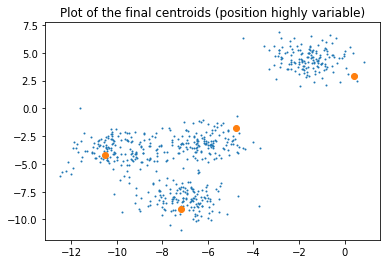### – labels

labels are the n integers that inform which cluster a datapoint belongs to

In :
labels = results_KbMOM['labels']
labels

Out:
array([3, 0, 3, 2, 1, 0, 0, 1, 0, 2, 1, 3, 0, 0, 1, 1, 2, 3, 1, 1, 3, 2,
1, 3, 1, 3, 3, 3, 1, 2, 1, 3, 1, 1, 3, 1, 2, 3, 0, 0, 1, 1, 3, 1,
1, 2, 2, 1, 2, 1, 0, 3, 0, 0, 0, 3, 1, 2, 1, 2, 2, 2, 3, 2, 3, 2,
1, 1, 2, 2, 3, 0, 0, 3, 3, 0, 3, 3, 1, 3, 2, 2, 3, 1, 2, 2, 2, 3,
1, 2, 1, 2, 0, 0, 3, 2, 2, 2, 1, 3, 2, 2, 0, 1, 2, 0, 3, 2, 1, 2,
2, 3, 1, 2, 3, 0, 3, 1, 1, 2, 1, 2, 2, 1, 0, 0, 3, 3, 0, 0, 2, 3,
3, 3, 3, 0, 0, 2, 1, 1, 0, 0, 3, 2, 1, 1, 3, 2, 1, 0, 2, 0, 2, 0,
3, 3, 2, 2, 2, 3, 2, 1, 2, 0, 0, 1, 1, 3, 2, 3, 1, 2, 3, 2, 2, 3,
3, 1, 1, 0, 1, 3, 2, 3, 2, 2, 2, 3, 2, 0, 1, 1, 1, 3, 0, 3, 1, 0,
1, 2, 1, 3, 1, 1, 3, 2, 3, 1, 3, 2, 3, 2, 1, 1, 3, 0, 1, 0, 0, 3,
3, 0, 0, 2, 1, 2, 3, 2, 2, 3, 3, 2, 3, 0, 2, 1, 1, 3, 0, 0, 3, 2,
2, 2, 2, 3, 1, 0, 2, 3, 2, 0, 2, 3, 0, 2, 2, 3, 1, 2, 1, 1, 0, 0,
3, 2, 1, 3, 0, 1, 0, 2, 2, 1, 0, 1, 3, 2, 0, 0, 1, 2, 3, 2, 3, 2,
3, 1, 1, 1, 1, 2, 0, 3, 1, 3, 2, 0, 0, 1, 3, 1, 3, 0, 2, 0, 3, 3,
1, 2, 3, 1, 1, 2, 2, 3, 3, 1, 1, 2, 3, 0, 0, 3, 3, 0, 2, 3, 2, 1,
0, 0, 1, 2, 3, 3, 0, 0, 3, 3, 3, 0, 3, 2, 2, 1, 0, 2, 0, 3, 1, 3,
3, 2, 0, 1, 0, 2, 0, 2, 0, 1, 3, 2, 2, 1, 3, 1, 0, 1, 2, 2, 0, 1,
2, 1, 0, 1, 1, 2, 2, 3, 0, 0, 2, 1, 3, 0, 1, 2, 3, 0, 2, 2, 0, 2,
3, 3, 2, 3, 1, 1, 1, 3, 2, 1, 2, 0, 3, 1, 0, 1, 2, 1, 3, 3, 2, 1,
1, 0, 0, 3, 0, 1, 3, 2, 2, 2, 3, 0, 3, 2, 0, 3, 1, 1, 1, 0, 3, 0,
3, 1, 3, 1, 1, 2, 2, 1, 1, 1, 0, 2, 2, 3, 2, 1, 2, 2, 0, 0, 2, 1,
3, 0, 1, 2, 0, 1, 1, 2, 2, 1, 3, 3, 2, 3, 3, 1, 1, 3, 2, 1, 0, 0,
2, 0, 2, 2, 0, 1, 1, 0, 0, 1, 0, 2, 3, 2, 0, 1, 0, 2, 2, 0, 3, 3,
2, 3, 3, 2, 0, 0, 2, 1, 1, 2, 3, 0, 0, 1, 3, 2, 3, 2, 2, 3, 3, 0,
3, 0, 0, 2, 2, 3, 0, 3, 0, 2, 2, 1, 2, 0, 2, 2, 0, 3, 0, 0, 3, 0,
2, 0, 3, 1, 1, 2, 1, 0, 2, 0, 2, 1, 1, 2, 0, 3, 3, 2, 2, 0, 3, 0,
0, 1, 3, 3, 2, 2, 3, 0, 1, 2, 0, 3, 1, 1, 2, 1, 3, 2, 0, 1, 0, 2,
0, 1, 1, 3, 1, 1])
In :
plt.scatter(X[:,0],X[:,1],color=plt.get_cmap('tab10')(labels))
plt.title('Plot of the final partition')
plt.show()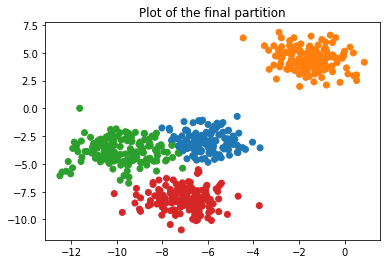### – id_Qblock_init

id_Qblock_init is the composition of the initial block that achieved the median value of the empirical risk among all blocks.

Qblock stands for « quantile block » because taking the median is not compulsory. It is possible to take another quantile.

In :
results_KbMOM['id_Qblock_init']

Out:
[[368, 8, 582, 530, 581, 581, 45, 164],
[28, 15, 207, 318],
[156, 431, 231, 241, 102, 427, 156],
]
In :
plt.scatter(X[:,0],X[:,1],s=3,alpha=0.5)
for num,cluster_sample in enumerate(results_KbMOM['id_Qblock_init']):
plt.scatter(X[cluster_sample,0],X[cluster_sample,1],color=plt.get_cmap('tab10')(num+1))
plt.title('Composition of the initial selected block \n One color for each intial cluster')
plt.show()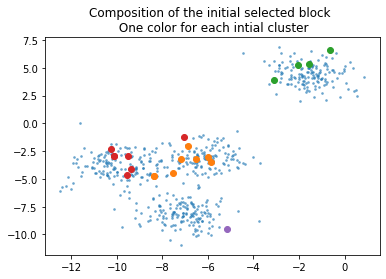### – convergence

convergence returns the list of all Aitkens criterion values. The first 2 values are None because Aitkens is based on the two previous quantities.

In :
results_KbMOM['convergence']

Out:
[None,
None,
1.654389994652857,
1.6724332851540002,
1.6098389737594274,
1.5780469038491978,
1.642370690499925,
1.5862243066642197,
1.6702825273687074,
1.6303510797913323,
1.7282894321499624]

### – scores

scores are the quantities that mimics a data depth. The lower the score of a datapoint, the further from all centroids along all iterations, the more the datapoint is an outlier in its cluster.

In :
scores = results_KbMOM['scores']
np.round(scores,2)

Out:
array([4.81, 3.05, 3.34, 4.56, 2.04, 4.42, 3.61, 2.83, 3.73, 5.45, 2.5 ,
5.46, 1.99, 4.24, 2.23, 3.78, 3.85, 6.32, 2.54, 1.86, 3.21, 4.04,
1.81, 5.05, 2.04, 4.02, 4.07, 3.63, 3.12, 5.42, 2.63, 2.34, 3.42,
1.84, 3.99, 2.18, 0.76, 4.71, 1.3 , 1.89, 1.52, 1.87, 3.29, 2.37,
2.17, 2.23, 4.57, 2.64, 3.51, 2.59, 1.8 , 4.58, 1.68, 3.57, 1.97,
3.15, 3.58, 2.8 , 2.28, 3.95, 3.88, 3.26, 5.45, 4.27, 1.84, 1.87,
4.55, 3.21, 1.39, 1.51, 4.52, 3.94, 1.79, 4.76, 4.66, 3.09, 3.64,
3.99, 3.19, 3.13, 2.1 , 2.72, 3.18, 3.56, 2.81, 3.43, 3.01, 4.45,
3.47, 3.73, 3.64, 2.12, 2.07, 2.03, 3.96, 3.28, 2.58, 3.72, 2.25,
4.36, 4.21, 2.73, 1.55, 2.24, 3.07, 4.69, 3.53, 1.  , 1.49, 3.77,
2.78, 4.76, 2.26, 3.05, 4.1 , 0.95, 4.97, 2.25, 3.84, 3.43, 3.07,
3.3 , 4.6 , 1.39, 2.57, 2.42, 2.35, 3.85, 1.15, 2.66, 2.16, 4.72,
4.04, 4.76, 3.1 , 1.76, 4.62, 2.93, 3.39, 1.63, 2.05, 2.98, 3.41,
2.94, 1.98, 3.79, 1.38, 1.92, 3.46, 3.56, 2.85, 3.01, 2.66, 1.11,
4.26, 3.63, 5.02, 3.08, 3.87, 4.44, 3.67, 2.27, 2.07, 0.48, 4.25,
1.95, 2.8 , 4.38, 3.31, 4.  , 3.84, 4.29, 5.01, 3.87, 2.76, 4.98,
5.41, 2.18, 1.7 , 3.51, 3.68, 4.18, 3.48, 4.25, 3.85, 3.01, 1.44,
3.31, 4.87, 0.96, 2.64, 1.93, 2.35, 3.16, 1.43, 2.74, 3.62, 2.04,
2.44, 1.64, 1.93, 4.18, 2.48, 1.15, 4.27, 2.52, 4.93, 4.16, 3.88,
3.42, 5.49, 3.48, 1.9 , 2.11, 3.16, 3.24, 2.51, 0.93, 2.84, 1.88,
4.91, 2.1 , 2.64, 0.86, 3.8 , 2.37, 1.55, 3.83, 2.68, 4.2 , 4.33,
6.88, 4.24, 3.49, 2.58, 1.92, 1.41, 3.59, 2.2 , 1.56, 5.01, 3.5 ,
2.97, 2.98, 2.58, 4.16, 2.52, 2.13, 3.62, 4.43, 3.64, 3.95, 1.85,
3.57, 4.65, 3.02, 2.86, 5.77, 2.55, 3.3 , 1.78, 1.91, 4.22, 2.5 ,
4.21, 3.84, 2.56, 3.46, 1.83, 1.74, 1.28, 4.39, 3.83, 1.18, 2.17,
2.88, 4.69, 1.8 , 3.64, 1.82, 2.2 , 3.96, 4.09, 3.83, 3.69, 3.84,
3.43, 2.89, 3.43, 2.06, 3.46, 1.64, 3.02, 2.26, 2.76, 3.74, 5.18,
4.25, 2.7 , 2.9 , 2.34, 3.5 , 3.77, 1.31, 5.18, 1.56, 2.18, 2.55,
4.42, 3.06, 4.09, 3.91, 2.98, 1.93, 3.4 , 1.33, 3.82, 2.5 , 2.24,
2.46, 3.44, 4.76, 2.52, 3.91, 4.74, 1.65, 3.  , 3.08, 4.27, 1.95,
3.41, 2.21, 1.1 , 2.73, 4.7 , 3.71, 4.16, 4.62, 3.65, 4.26, 4.54,
1.76, 4.18, 3.91, 2.75, 1.76, 4.93, 0.92, 4.53, 3.8 , 1.87, 3.46,
4.74, 2.83, 1.25, 1.72, 3.  , 0.14, 3.76, 2.77, 1.22, 3.09, 4.71,
5.49, 4.14, 4.44, 5.16, 1.59, 4.39, 2.09, 1.91, 1.98, 3.23, 2.49,
3.07, 1.44, 2.62, 2.47, 2.03, 1.01, 4.38, 4.82, 2.74, 2.46, 1.39,
3.07, 4.73, 2.81, 4.  , 2.66, 2.06, 3.36, 3.19, 2.98, 2.73, 2.67,
4.87, 2.9 , 2.73, 1.48, 1.05, 1.46, 1.91, 2.28, 2.44, 2.  , 2.22,
2.93, 4.65, 1.32, 0.64, 1.96, 2.47, 2.71, 2.38, 3.51, 2.3 , 3.56,
2.26, 2.61, 4.2 , 4.03, 1.45, 2.37, 2.43, 3.1 , 4.37, 3.1 , 5.14,
2.83, 5.44, 3.59, 1.1 , 4.27, 3.78, 2.44, 4.7 , 3.4 , 4.69, 1.41,
4.97, 1.19, 1.78, 2.23, 3.37, 2.87, 3.02, 1.08, 3.57, 1.33, 1.57,
2.96, 2.45, 4.18, 4.25, 2.7 , 3.22, 5.47, 1.93, 4.77, 2.92, 3.52,
3.13, 2.15, 2.43, 3.54, 2.46, 1.2 , 2.55, 6.69, 3.13, 3.64, 3.27,
1.17, 3.66, 2.15, 4.69, 2.64, 3.54, 4.59, 0.98, 3.7 , 1.27, 2.83,
3.87, 1.32, 2.79, 2.4 , 4.29, 3.35, 1.35, 2.04, 1.39, 2.19, 2.61,
3.39, 3.72, 4.47, 4.18, 3.93, 0.72, 4.15, 3.57, 5.02, 2.48, 4.42,
5.21, 2.89, 4.08, 3.67, 1.57, 1.99, 2.25, 2.99, 1.04, 2.77, 3.84,
2.01, 2.35, 2.05, 3.2 , 3.19, 4.04, 3.  , 3.8 , 3.63, 4.23, 3.3 ,
4.05, 3.55, 4.62, 4.55, 2.18, 4.27, 1.41, 3.67, 2.01, 4.95, 3.75,
2.64, 2.93, 1.93, 2.06, 2.06, 1.71, 4.89, 4.73, 3.65, 3.9 , 3.59,
3.08, 2.35, 3.38, 1.94, 1.81, 3.55, 3.04, 1.51, 2.68, 4.29, 3.87,
3.41, 3.66, 1.56, 2.68, 3.54, 3.87, 3.66, 3.72, 4.14, 1.46, 2.44,
4.67, 1.57, 3.64, 2.8 , 0.62, 3.01, 3.52, 1.88, 2.58, 3.02, 2.73,
0.52, 2.4 , 1.7 , 2.49, 2.51, 4.02, 2.57, 1.65, 2.39, 3.51, 3.48,
3.42, 2.53, 3.43, 2.27, 3.79, 3.01])
In :
# as shown on the plot, the greatest datapoints are the point whose scores are lowest
# and it seems to coincide with a data depth
# warning /!\ the sizes on the plot are not proportionnal to scores
plt.scatter(X[:,0],X[:,1],color=plt.get_cmap('tab10')(labels),s=1/scores**3)
plt.title('The size of the points are related to their \n score (data-depth like value)')
plt.show()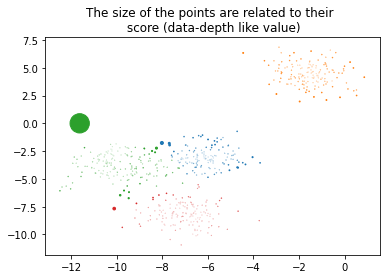### – Qb_risk

Qb_risk is the list of all values encountered for the median risk throughout iterations.

As one can see, the risk of the median bloc is not monotonous. The algorithm has though good empirical performances (Qb_block stands for « quantile block » because one can choose another quantile than median)

In :
results_KbMOM['Qb_risk']

Out:
[1.7500958670003555,
1.6694033275691018,
1.6567451287708095,
1.591205002642679,
1.6172415178284425,
1.6947976433818026,
1.5329924222850775,
1.6123231040321695,
1.645813941293566,
1.6170883547338206,
1.5783578647217495]

### – Qb_centers

Qb_centers is simply the list of all sets of centroids encountered at each steps of the algorithm

Qb stands for « quantile block » because taking the median is not compulsory. It is possible to take another quantile.

In :
Qb_centers = results_KbMOM['Qb_centers']
Qb_centers

Out:
[array([[-7.10057907, -3.61362142],
[-1.83724548,  5.26348224],
[-9.43803981, -3.00970852],
[-5.1450899 , -9.54492198]]), array([[ -5.98287145,  -3.09665204],
[ -2.27986446,   4.84117782],
[-10.18453369,  -3.16584064],
[ -7.49531724,  -8.13852482]]), array([[ -6.00059938,  -3.51085505],
[ -1.15395686,   4.39244479],
[-10.09783712,  -3.5665143 ],
[ -6.78742338,  -8.38369569]]), array([[ -6.11781281,  -2.91514716],
[ -1.41984143,   4.78518195],
[-10.34678315,  -4.05487162],
[ -6.87361637,  -8.17011308]]), array([[ -6.93595347,  -3.20166746],
[ -1.09694799,   4.5273377 ],
[-10.4909522 ,  -4.42985701],
[ -6.76436449,  -8.15928559]]), array([[-5.92732468, -3.23120499],
[-2.03038093,  4.75705775],
[-9.9075231 , -2.77895483],
[-6.76082864, -8.30484026]]), array([[ -6.7744647 ,  -2.81848195],
[ -1.45193924,   4.56236768],
[-10.70093523,  -3.54054043],
[ -6.58010992,  -7.96033569]]), array([[ -5.91148393,  -3.26641543],
[ -2.13653695,   4.93064359],
[-10.98031794,  -3.69922651],
[ -6.5357437 ,  -8.2276136 ]]), array([[ -5.76159888,  -3.28659784],
[ -1.38706787,   4.34104325],
[-10.19400979,  -3.7545838 ],
[ -7.2089274 ,  -8.0850791 ]]), array([[ -5.9133541 ,  -3.12224564],
[ -1.17910706,   4.37754903],
[-10.66098972,  -3.38147444],
[ -6.99804748,  -8.31253364]]), array([[ -4.76718795,  -1.74560175],
[  0.40622341,   2.93533294],
[-10.53661635,  -4.21951699],
[ -7.20441682,  -9.07633508]])]
In :
plt.scatter(X[:,0],X[:,1],1)
for num,centroids in enumerate(Qb_centers):
plt.scatter(centroids[:,0],centroids[:,1],color=plt.get_cmap('tab10')(1))
plt.title('Trace of all encountered centroids')
plt.show()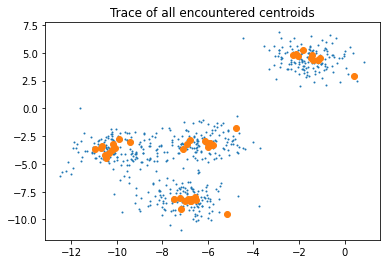In :
ncols=2
nb_graphs = len(Qb_centers)
nrows=int(nb_graphs/ncols+1)
size_1_plot = 5

fig,axes = plt.subplots(ncols=ncols,nrows=nrows,figsize=(size_1_plot*ncols,size_1_plot*nrows ))
for num,centroids in enumerate(Qb_centers):
axes[int(num/ncols),num%ncols].scatter(X[:,0],X[:,1],s=3,alpha=0.5)
axes[int(num/ncols),num%ncols].scatter(centroids[:,0],centroids[:,1],color=plt.get_cmap('tab10')(1),s=50)
axes[int(num/ncols),num%ncols].set_title(f'Centroids at iteration number {num}')
for num_graph in range(ncols*nrows):
if nb_graphs <= num_graph:
axes[int(num_graph/ncols),num_graph%ncols].axis('off')
st = fig.suptitle('All sets of centroids throughout the algorithm', fontsize=20)
st.set_y(0.9)
plt.show()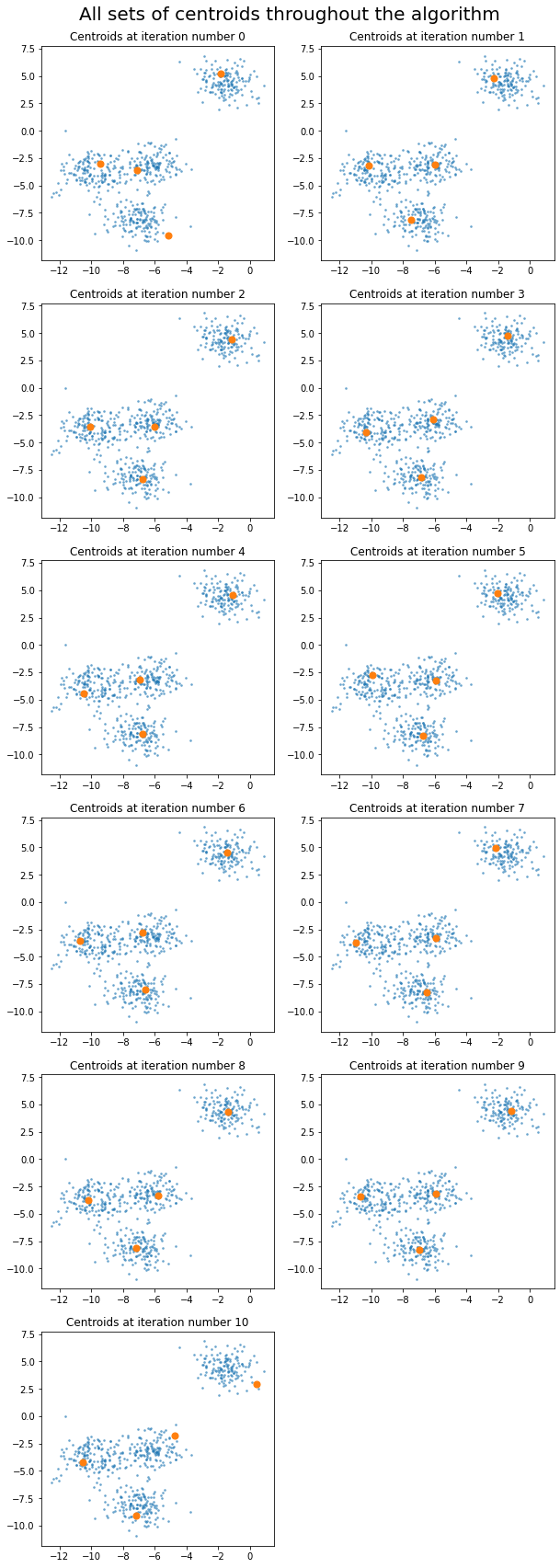### – B

B is the number of block created a each step of the algorithm

In :
results_KbMOM['B']

Out:
15

### – t

t is the size of all created blocks

In :
results_KbMOM['t']

Out:
20